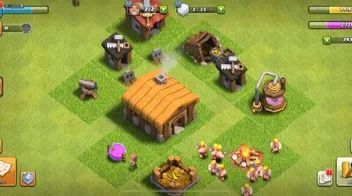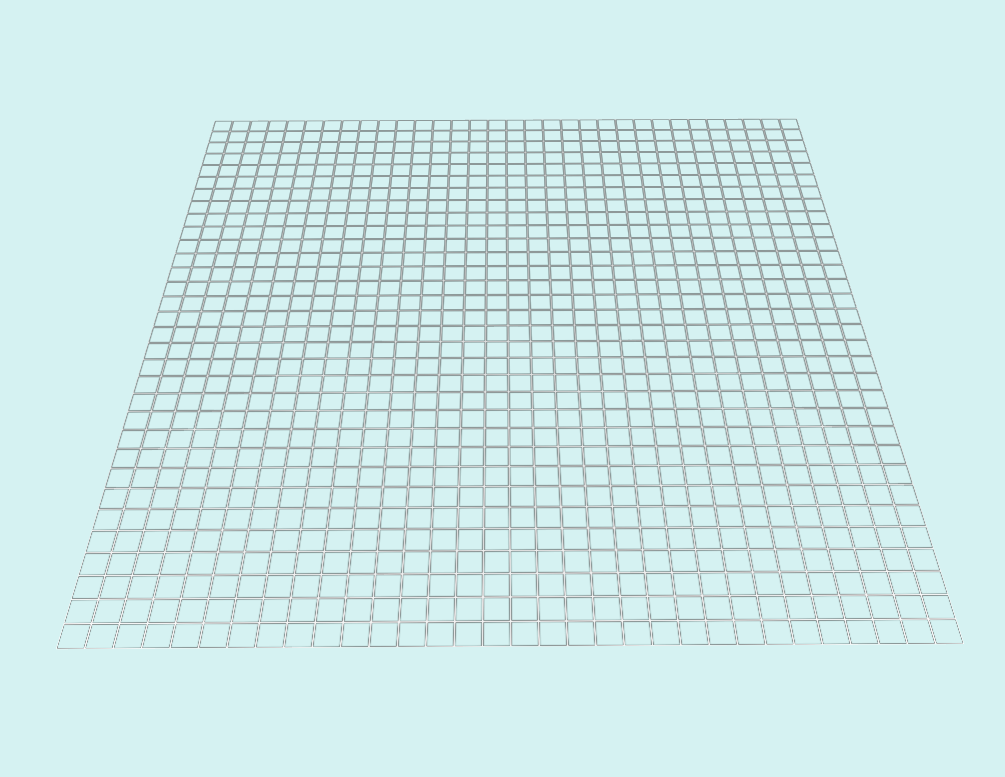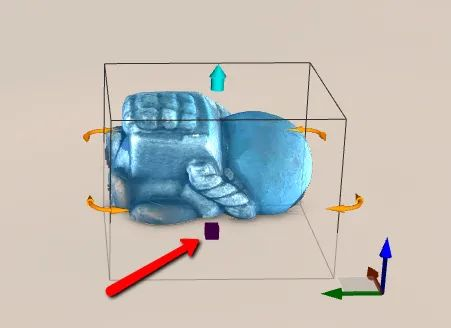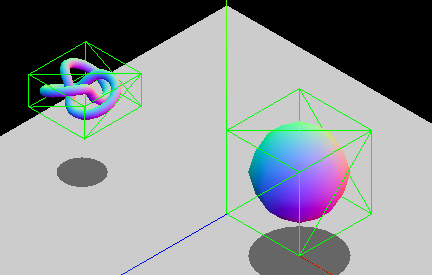# 3D 沙盒游戏之地面网格设计

2022/04/14 18:00

## 背景—## 目标—

1. 完成地面初始化，且可以改变特定位置的纹理
2. 获取模型在地面上的位置信息

## 实现之地面创建篇—

//地面的长度（x方向）const GROUND_WIDTH = 64//地面的宽度（z方向const GROUND_HEIGHT = 64//地面纹理的宽度 const TEXTURE_WIDTH = 1024//地面纹理的高度const TEXTURE_HEIGHT = 1024//一个方向上（s和t坐标方向），把地面分为多少个小块const GROUND_SUBDIVISION = 32

### 1. 建立一块平地

const ground = MeshBuilder.CreateGround(    name,    { width: GROUND_WIDTH, height: GROUND_HEIGHT, subdivisions: GROUND_SUBDIVISION },    scene,)

### 2. 给“大地”贴上纹理

//创建一个标准材质const groundMaterial = new StandardMaterial('groundMaterial', scene);//创建一个纹理const groundTexture = new Texture('//storage.360buyimg.com/model-rendering-tool/files/jdLife/grass.jpg', scene)//把纹理赋值给材质的漫反射纹理（这里也可以是其他类型的纹理）groundMaterial.diffuseTexture = groundTexture//把材质赋值给地面的材质属性ground.material = groundMaterial

### 3. 分割地面为一个个格子

1. 使用了 babylon.js 的 AdvancedDynamicTexture.CreateForMesh高级动态纹理为地面创建纹理。
3. “容器”是 Babylon.js 的一个类 Container。

//首先调用AdvancedDynamicTexture的api，创建纹理const groundTexture = GUI.AdvancedDynamicTexture.CreateForMesh(ground, TEXTURE_WIDTH, TEXTURE_HEIGHT, false)//创建最外层的Container -- panel，他的宽高和纹理的一致const panel = new GUI.StackPanel()panel.height = TEXTURE_HEIGHT + 'px'panel.width = TEXTURE_WIDTH + 'px'//把panel添加到纹理上groundTexture.addControl(panel)//循环的建立一列列Row，并且加到panel上面for (let i = 0; i < GROUND_SUBDIVISION; i++) {  const row = new GUI.StackPanel()  row.height = TEXTURE_HEIGHT / GROUND_SUBDIVISION + 'px'  //把row添加到panel上  panel.addControl(row)  row.isVertical = false  //在循环的，在每一行里面建立一个个格子  for (let j = 0; j < GROUND_SUBDIVISION; j++) {    const block = new GUI.Rectangle()    block.width = TEXTURE_WIDTH / GROUND_SUBDIVISION + 'px'    row.addControl(block)       }}### 4. 给每个格子单独贴图，并且存储纹理 Image 对象

...  //在循环的，在每一行里面建立一个个格子  for (let j = 0; j < GROUND_SUBDIVISION; j++) {    const block = new GUI.Rectangle()    block.width = TEXTURE_WIDTH / GROUND_SUBDIVISION + 'px'    row.addControl(block)    //隐藏格子的边框    block.thickness = 0    //创建Image对象    const blockImage = new GUI.Image('blockTexture','//storage.360buyimg.com/model-rendering-tool/files/jdLife/grass.jpg')    //把图片添加到block上    block.addControl(blockImage)    //在外面的定义域里面先创建好blockImageArray    blockImageArray.push(blockImage)  }

//把需要的图片导入好，放到imageSource里面const imageSource : { [key: string]: HTMLImageElement } = { grass: img, stone: img }...//创建image的时候不再传递url参数了const blockImage = new GUI.Image()//对domImage属性赋值blockImage.domImage = imageSource.grass

### 5. 更改纹理

panel.onPointerClickObservable.add(e => {    const { y, x } = e    const perBlockWidth = TEXTURE_WIDTH / GROUND_SUBDIVISION    const perBlockHeight = TEXTURE_HEIGHT / GROUND_SUBDIVISION    const row = Math.floor(y / perBlockHeight)    const col = Math.floor(x / perBlockWidth)    const index = row * GROUND_SUBDIVISION + col    blockObjArr[index].domImage = imageSource.stone})

## 实现之模型占位计算篇—

• 地面：案例中的平面
• 模型：案例中需要计算占位的物体
• 索引编号：二维数组的下标
• 网格坐标系：将地面分割为均等网格而形成的坐标系
• WebGL 坐标系：原始 WebGL 坐标系
• 模型基点：模型原点
• 转换：从一个值换算到另一个值
• 纠偏：把原有的坐标数值加上偏正值（这里是半个格子的长度或宽度）
• 包围盒：能把模型整个包起来的最小长方体 bounding

//重新定义一下，让地面分为 8 * 8 的网格const GROUND_SUBDIVISION = 8//每一个格子在相机裁剪坐标系中的宽度const PER_BLOCK_VEC_X = GROUND_WIDTH / GROUND_SUBDIVISION//每一个格子在相机裁剪坐标系中的高度const PER_BLOCK_VEC_Z = GROUND_HEIGHT / GROUND_SUBDIVISION//模型位置向量在x轴方向的偏移量const CENTER_OFF_X = PER_BLOCK_VEC_X / 2//模型位置向量在z轴方向的偏移量const CENTER_OFF_Z = PER_BLOCK_VEC_Z / 2//半个格子在相机裁剪坐标系中的宽度const HALF_BLOCK_VEC_X = PER_BLOCK_VEC_X / 2//半一个格子在相机裁剪坐标系中的高度const HALF_BLOCK_VEC_Z = PER_BLOCK_VEC_Z / 2

for (let i = 0; i < GROUND_SUBDIVISION; i++) {  const row = new GUI.StackPanel()  row.height = TEXTURE_HEIGHT / GROUND_SUBDIVISION + 'px'  //把row添加到panel上  panel.addControl(row)  row.isVertical = false  //在循环的，在每一行里面建立一个个格子  for (let j = 0; j < GROUND_SUBDIVISION; j++) {    const block = new GUI.Rectangle()    block.width = TEXTURE_WIDTH / GROUND_SUBDIVISION + 'px'    row.addControl(block)         //创建贴图    const blockImage = new GUI.Image()    //对domImage属性赋值    blockImage.domImage = imageSource.grass    block.addControl(blockImage)    blockImageArray.push(blockImage)  }}

interface Coord {  x: number,  z: number} 

### 1. 创建网格坐标系坐标集合数组：groundStatus

groundStatus数据结构如下：

type GroundStatus = number[][]

### 2. 模型基点向量与网格坐标系坐标的换算WebGL 坐标系的 (0, 0, 0) 换算成地面坐标系，就是网格坐标 (3, 3)、(3, 4)、(4, 3)、(4, 4) 这四个格子的交点。如下图所示：

function getGroundCoordByModelPos(buildPosition: Vector3): Coord {  const { _x, _z } = buildPosition  const coordX = Math.floor(GROUND_WIDTH / 2 / PER_BLOCK_VEC_X + (_x + CENTER_OFF_X) / PER_BLOCK_VEC_X)  const coordZ = Math.floor((GROUND_HEIGHT / 2 - (_z - CENTER_OFF_Z)) / PER_BLOCK_VEC_Z)  return { x: coordX, y: coordZ }}

### 3. 获取模型占位区在 WebGL 坐标系的关键数据interface RawOffsetMap {  rawOffsetTop: number  rawOffsetBottom: number  rawOffsetLeft: number  rawOffsetRight: number}

/* @param { AbstractMesh[] } meshes 模型导入后返回的结果 @param { Vector3 } scale 模型的缩放倍数*/function getRawOffsetMap(meshes: AbstractMesh[], scale: Vector3 = new Vector3(1, 1, 1)): RawOffsetMap {  //声明最小的向量  let min = null  //声明最大的向量  let max = null    //对模型的meshes数组进行遍历  meshes.forEach(function (mesh) {    //babylon.js 提供的api，可以遍历该mesh的和mesh的所有子mesh，找到它们的边界    const boundingBox = mesh.getHierarchyBoundingVectors()    //如果当前的最小向量不存在，那么把当前的mesh的boundingBox的min属性赋值给它    if (min === null) {      min = new Vector3()      min.copyFrom(boundingBox.min)    }    //如果当前的最大向量不存在，那么把当前的mesh的boundingBox的max属性赋值给它    if (max === null) {      max = new Vector3()      max.copyFrom(boundingBox.max)    }    //对最小向量和当前的boundingBox的min属性，从x，y，z这三个分量进行比较与再赋值    min.x = boundingBox.min.x < min.x ? boundingBox.min.x : min.x    min.y = boundingBox.min.y < min.y ? boundingBox.min.y : min.y    min.z = boundingBox.min.z < min.z ? boundingBox.min.z : min.z    //对最大向量和当前的boundingBox的max属性，从x，y，z这三个分量进行比较与再赋值    max.x = boundingBox.max.x > max.x ? boundingBox.max.x : max.x    max.y = boundingBox.max.y > max.y ? boundingBox.max.y : max.y    max.z = boundingBox.max.z > max.z ? boundingBox.max.z : max.z  })  return {    rawOffsetRight: max.x * scale.x,    rawOffsetLeft: Math.abs(min.x * scale.x),    rawOffsetBottom: max.z * scale.z,    rawOffsetTop: Math.abs(min.z * scale.z)  }}

### 4. 获取模型占位区在网格坐标系上的关键数据：offsetMap

interface OffsetMap {  offsetLeft: number,  offsetRight: number,  offsetTop: number,  offsetBottom: number} 

function getModelOffsetMap(rawOffsetMap: RawOffsetMap): OffsetMap {  const { rawOffsetMapLeft, rawOffsetRight, rawOffsetBottom, rawOffsetTop } = rawOffsetMap  const offsetLeft = Math.ceil((rawOffsetLeft - HALF_BLOCK_VEC_X) / PER_BLOCK_VEC_X)  const offsetRight = Math.ceil((rawOffsetRight - HALF_BLOCK_VEC_X) / PER_BLOCK_VEC_X)  const offsetTop = Math.ceil((rawOffsetTop - HALF_BLOCK_VEC_Z) / PER_BLOCK_VEC_Z)  const offsetBottom = Math.ceil((rawOffsetBottom - HALF_BLOCK_VEC_Z) / PER_BLOCK_VEC_Z)  return {    offsetBottom,    offsetLeft,    offsetRight,    offsetTop  }}

### 5. 计算出模型在网格坐标系的包围盒索引：bounding

bounding 的数据结构如下：

interface Bounding {  minX: number,  maxX: number,  minZ: number,  maxZ: number} 

function getModelBounding(buildPosition: Vector3, offsetMap: OffsetMap): IBounding {  const modelGroundPosCoord = getGroundCoordByModelPos(buildPosition)  const { x, y } = modelGroundPosCoord  const { offsetBottom, offsetLeft, offsetRight, offsetTop } = offsetMap    const minX = x - offLeft  const maxX = x + offRight  const minZ = y - offTop  const maxZ = y + offBottom    return {    minX,    maxX,    minZ,    maxZ  }}

### 6. 更新占位数据

//索引边界判断function isValidIndex(x: number, z: number): boolean {  if (x >= 0 && x < GROUND_SUBDIVISION && z >= 0 && z < GROUND_SUBDIVISION) return true  return false}function setModlePosition(groundStatus: GroundStatus, bounding: Bounding) {  const { minX, maxX, minZ, maxZ } = bounding  for (let i = minZ; i <= maxZ; i++) {    for (let j = minX; j <= maxX; j++) {      if (isValidIndex(j, i))        groundStatus[i][j]++    }  }}

## 参考链接—



babylon.js 的相关文档: https://doc.babylonjs.com/typedoc#createground-2



WebGL坐标系基础: https://juejin.cn/post/6890795086054260750



babylon.js材质与纹理: https://babylonjsguide.github.io/basics/Materials





WebGL纹理详解之三：纹理尺寸与Mipmapping: http://www.jiazhengblog.com/blog/2016/01/05/2882/



Image : https://doc.babylonjs.com/typedoc/classes/babylon.gui.image







3D 碰撞检测: https://developer.mozilla.org/zh-CN/docs/Games/Techniques/3D_collision_detection



WebGL纹理详解之三：纹理尺寸与Mipmapping: http://www.jiazhengblog.com/blog/2016/01/05/2882/



WebGL坐标系基础: https://juejin.cn/post/6890795086054260750



babylon.js 官网: https://www.babylonjs.com/0 评论
19 收藏
6